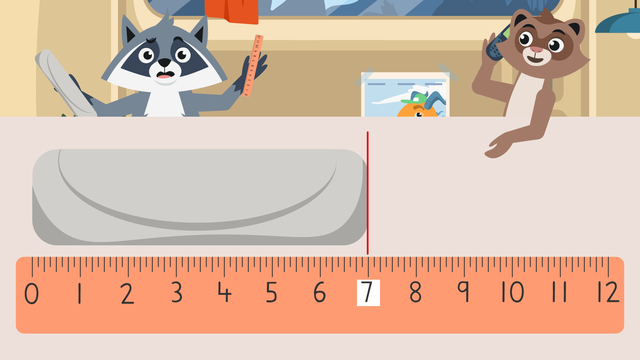# Measuring in Inches

Content Measuring in InchesRating

Ø 5.0 / 1 ratings
The authorsTeam Digital
Measuring in Inches
CCSS.MATH.CONTENT.2.MD.A.4

## Measuring Length in Inches

An inch is a small unit of measurement used in the United States to describe how long, wide, or tall something is. An inch is about the length of a quarter or a paperclip. It can be used to find the size of things like cell phones, laptops, trading cards, and food. Inch can be abbreviated with the letters I N period or with one set of quotation marks that follow the number.To find an object's size in inches, we can use a measuring ruler in inches to determine its length. Rulers are tools that give us precise, or exact, measurements in a given unit.## Measuring Lengths in Inches with Rulers

To practice measuring in inches with a ruler, let's use the order in the image below. First, line up one end of the food with the zero mark of the ruler.

Next, find the other end of the food and determine which mark it is closest to.Then, read the number at the inch mark to find the length of the object. Here it lines up with the number eight.Lastly, record the measurement by writing the number and labeling it in inches.## Measuring Inches – Summary

To measure using an inch ruler, you need to follow these steps.

Step # What to do
1 Line up one end of an object up
with the zero mark of the ruler.
2 Find the other end of the object and
determine which mark it is closest to.
3 Read the number at the inch mark
to find the length of the object.
4 Record the measurement by writing
the number and labeling it in inches.

Have you practiced with a 2nd grade measuring in inches worksheet yet? On this website, you can also find measuring length in inches worksheets and interactive exercises.

### TranscriptMeasuring in Inches

Freddie and Zuri decided to get sandwiches for lunch today. Zuri ordered the six inch Tuna Toastie. Freddie ordered the seven inch Mega Melt. They decided to split the eight inch spiral glazed donut. But when the delivery arrived, all the food was wrapped the same! Let's learn about "Measuring in Inches" to see if we can help Freddie and Zuri tell the orders apart! An inch is a small unit of measurement used in the United States to describe how long, wide, or tall something is. An inch is about the length of a quarter or a paperclip. It can be used to find the size of things like cell phones, laptops, trading cards, and food. Inch can be abbreviated with the letters I N period or with one set of quotation marks that follow the number. To find an object's size in inches, we can use an inch ruler to measure it. Rulers are tools that give us precise, or exact, measurements in a given unit. To practice measuring using an inch ruler, let's use this order. First, line up one end of the food with the zero mark of the ruler. Next, find the other end of the food and determine which mark it is closest to. Then, read the number at the inch mark to find the length of the object. Here it lines up with the number eight. Lastly, record the measurement by writing the number and labeling it inches. This must be the eight inch spiral glazed donut! Try measuring this sandwich now! First, line up one end of the sandwich with the zero mark of the ruler. Next, look at the other end of the sandwich and find the closest mark. Then, read the number to find the length of the sandwich. Here it lines up with the number seven! THIS must be the seven inch Mega Melt! Now we can measure the final sandwich! The first end of the sandwich is lined up with the zero mark of the ruler. What number mark is the other end of this sandwich lined up to? It is lined up to eleven. So this sandwich is eleven inches long. "Wait a second...the Tuna Toastie was supposed to be six inches!" Before we sort out the sandwich situation, let's remember! Today, we learned about measuring in inches. An inch is a small measurement used to determine how long, wide, or tall something is. To find an object's size in inches, we can use inch rulers to measure it exactly. To measure using an inch ruler: First, line up one end of an object up with the zero mark of the ruler. Next, find the other end of the object and determine which mark it is closest to. Then, read the number at the inch mark to find the length of the object. Lastly, record the measurement by writing the number and labeling it in inches. "Who could that be?" "It was the delivery driver....turns out that wasn’t our order." "That explains the eleven inch sandwich. I guess we'll have to measure them all over again." "Why don't we just open them this time?"

## Measuring in Inches exercise

Would you like to apply the knowledge you’ve learned? You can review and practice it with the tasks for the video Measuring in Inches.